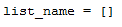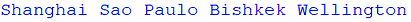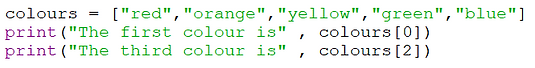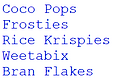top of page
top

## Python 8a - Using Lists## Lists

A list is a series of data in order

Data can be added to and removed from lists so they can change in size (unlike an array which is fixed and not used in Python).

It is important to note that each data element in a list has an index so that it can be specifically referenced (to delete it for example) and that indexes start at 0.

A list of the rainbow colours in order would start at 0 like this:## Set Up & Add to a List

To define a list and its contents, you need to declare the list name and use square brackets to hold its values.

An empty list could be defined as below:Now for a specific example for a geography app.

If you wanted to create a list with some data elements already inside, then you just need to add them within the square brackets and separate each one with a comma, such as:To add a new entry to the end of a list use the .append() command.

Write .append() after the name of your list, with the new data in brackets. For example:To print the list use an asterisk - * - before the list name otherwise it will print it with brackets and apostrophes.Create a list named bands and include three of your favourite musicians.

Use the .append command to add two more bands to your list.

Print the list by writing print(bands)

Example solution:## Remove Data from a List

There are two main ways of removing data from a list.

To delete data in a specific position in your list use the .pop() command, with the position in the brackets. For example:In the above example “Sao Paulo” would be removed from the list because it is second in the list (remember 0 is first, and 1 is second in Python).

Alternatively, if you want to delete data with a certain value use the .remove() command, with the value in brackets. For example:Create a list with five elements and print it.

Remove the first and last elements of the list and print it again.

Example solution:## Printing Lists

Type the list name into a print command to output the complete list. Typing an asterisk * before the list name removes punctuation:To print a list line-by-line use a for loop to cycle through each item.

'city' is just a variable name and can be replaced by anything relevant to the context, such as 'colour' in a list of colours or 'names' in a list of people.To print the data elements on the same line then you can use the end command which prevents Python from creating a new line and instead states what should go after each entry.

For example, end = “,  “  adds a comma and space between each element:To print an element with a certain index, put the index in square brackets. But remember that the index starts at 0 not 1.Create a list of at least five breakfast cereals.

Print each cereal on a separate line.

Then print just the first cereal in a message underneath, using its index.

Example solution:## Finding the Length

To find the length of a list use the len function.

You must put the name of the list inside brackets after the len command and save the answer into a variable.

For example:If you printed the length in the example above, by writing print(length), then the program would output: 4

If you wanted to print or delete the last value in a list, then you would need to change the value of the length variable by minus 1 (remember that if a list has 8 entries, then the last one will have the index 7, not 8).

For example, to pop the final entry of a list, you could write the following code:Ask the user to enter as many words as they can in 20 seconds.

Add each word to a list.

When the 20 seconds is up the user has to enter STOP. You do not need to count, the user should be trusted to type STOP.

Use the len function to print the first and last words entered - do not count STOP as an entered word.

Look back in 'Printing Lists' above at how to print an element using its index - remember to use square brackets, like cities.

Extra Challenge: Also print the total number of words entered.

Example solution:## Sorting Lists

The .sort() command will sort elements in a list into alphabetical order (if a string) or numerical order (if a number).The .sort() command can be used to sort values in descending order by including reverse = True in the brackets.Create a list of at least six names.

Sort the list.

Print each element on a separate line.

Example solution:## Searching Through Lists

An if statement can be used to see if a certain word appears within a list.Create a list of five of your friends.

Create an input line to ask a user to enter a name.

Use an if statement to check if their name is in the list.

Print appropriate responses if it is found and if it isn't.

Example solution:## Calculating the Sum of a List

To calculate the sum of a list of numbers there are two methods.

Using Python's built-in sum function:

numbers = [1,4,2,3,4,5]

print(sum(numbers))

Both methods will result in the same output:

19

Using a for loop to cycle through each number in the list and add it to a total.

numbers = [1,4,2,3,4,5]

total = 0
for number in numbers:
total = total + number

print(total)

Example solution:

Ask the user to input 5 numbers and append each to a list.

Use the sum command to output the total and the average.

Enter a number: 6

Enter a number: 7

Enter a number: 6

Enter a number: 9

Enter a number: 4

The total is 32

The average is 6.4

## Extending a List

.extend() can be used in a similar way to .append() that adds iterable items to the end of a list.

This commands works well with the choice command (imported from the random library) to create a list of characters that can be randomly selected.

The code below adds a lowercase alphabet to an empty list and then, depending on the choice of the user, adds an uppercase alphabet too. The choice command is used in a loop to randomly select 5 characters.

Using .extend() to make a random 5-character code

from random import choice

list = []

list.extend("abcdefghijklmnopqrstuvwxyz")

upper = input("Include uppercase letters? ")
if upper == "yes":

list.extend("ABCDEFGHIJKLMNOPQRSTUVWXYZ")

code = ""
for number in range(5):
letter =
choice(list)
code = code + letter

print("Your five character code is" , code)

Possible outputs:

Include uppercase letters? yes

Your five character code is yPfRe

Include uppercase letters? yes

Your five character code is GJuQw

=

Include uppercase letters? no

Your five character code is gberv

Extend treats each character as an indidual item whereas append adds the whole string as a single entity.

Most of time append would be used, but extend is suitable for a password program as additional individual characters can be added to a list depending on the parameters (e.g. lowercase letters, uppercase letters, numbers and special characters).

list = []

list.extend("ABCD")

list.extend("EFGH")

print(list)

list = []

list.append("ABCD")

list.append("EFGH")

print(list)

['A','B','C','D','E','F','G','H']

['ABCD' , 'EFGH']

=

=

Ask the user if they want uppercase letters, numbers and special characters  and use the extend command to add them to a list of characters if they type yes (you should extend lowercase characters into an empty list regardless, like in the code above).

Use a for loop and the choice command (imported from the random library) to randomly generate a 10-character password.

Example solutions:

Include uppercase letters? yes
Include numbers? yes
Include special characters? yes